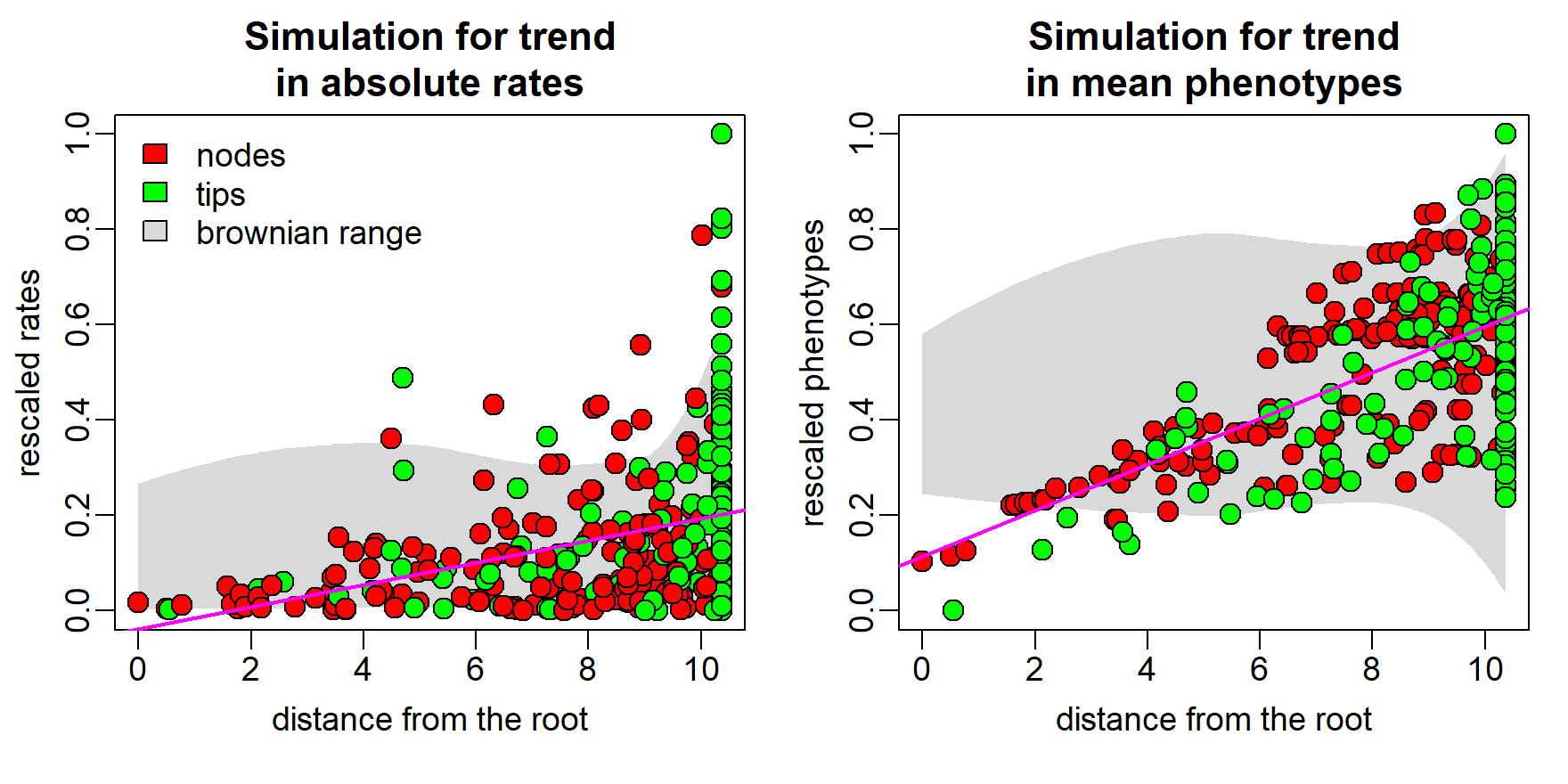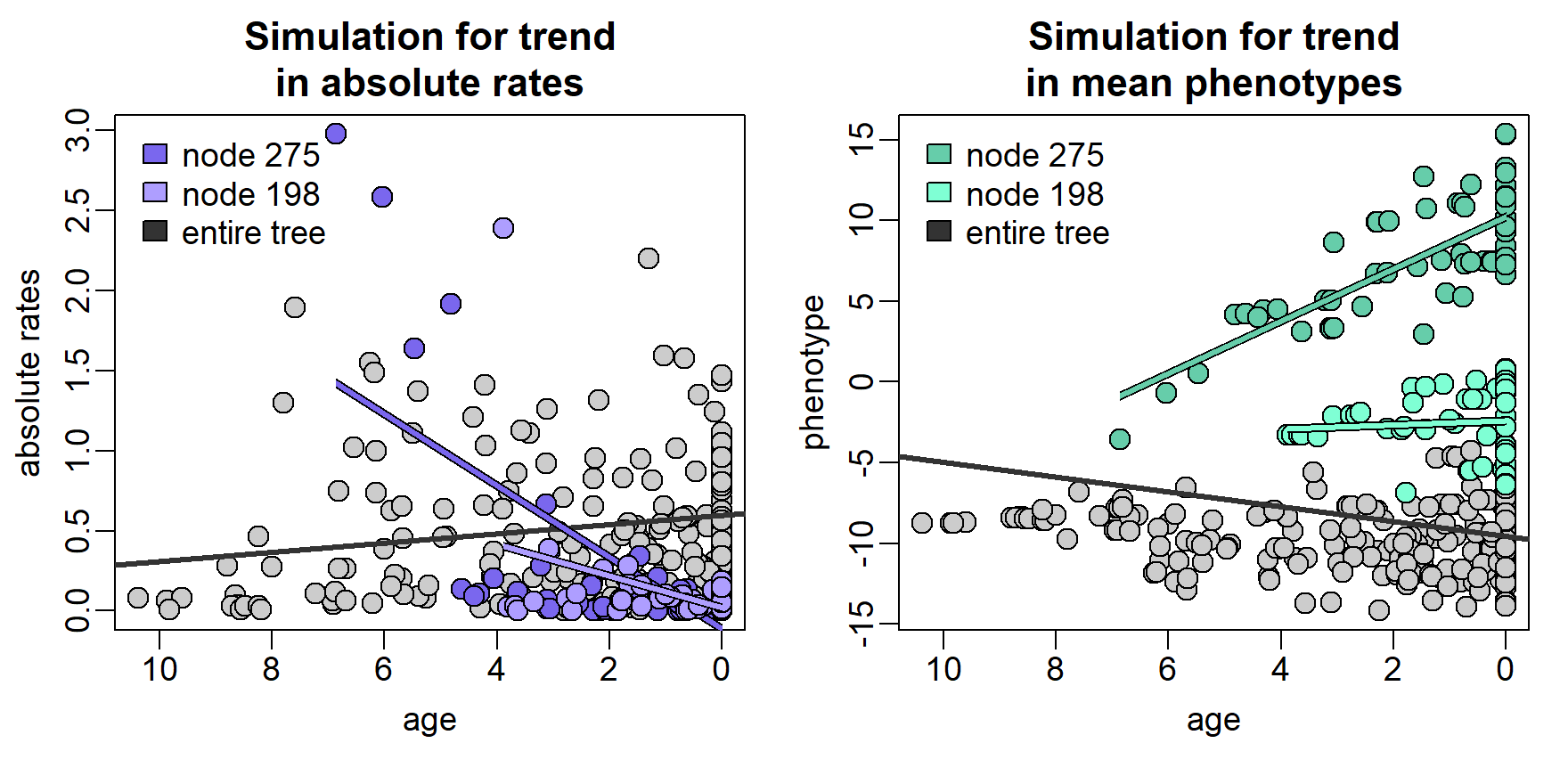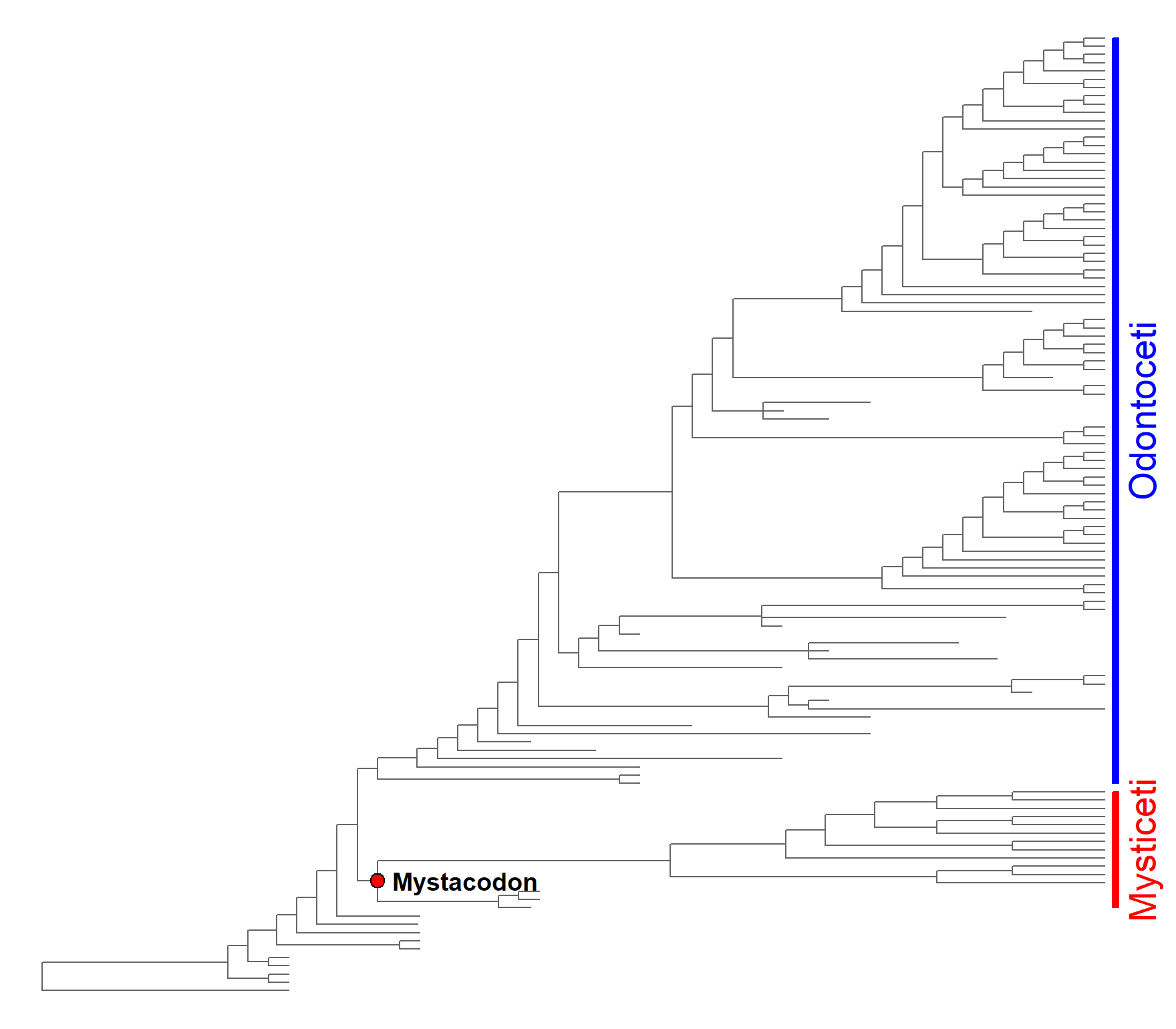# Searching for temporal trends in phenotypes or rates

## search.trend basics

The branch-wise estimation of phenotypic evolutionary rates and the computation of ancestral states at each node make RRphylo especially suitable to study temporal trends in phenotypic means and absolute rates applying to the entire phylogeny or just on a part of it. This is particularly true as the RRphylo method is specifically meant to work with phylogenies of extinct species, taking full advantage from fossil information. The function search.trend (Castiglione et al. 2019) is designed to explore the domain of macroevolution, addressing patterns such as Cope’s rule, or to test other specific research questions as to whether the rate of evolution increased/decreased in a particular tree or clade within it. Although possible in principle, running search.trend without extinct species in the tree makes little sense.

search.trend takes an object produced by RRphylo, which is necessary to retrieve branch-wise rate estimates and ancestral characters at nodes. By default, the function searches for significant temporal trends in phenotypic means and absolute evolutionary rates as applying to the entire phylogeny. If a specific hypothesis about one or more clades is tested, the clades presumed to experience trended evolution, in either phenotypes, rates or both, must be indicated by the argument node. If more than one clade is under testing, the function performs a pairwise comparison of trends also between the clades.

## Temporal trends on the entire tree

To search for temporal trends in either phenotypes or absolute rates occurring on the entire phylogeny, the function computes the regression slopes between phenotypic (absolute rate) values at nodes and tips and their age, meant as the distance from the tree root. Since a significant relationship between phenotypes (absolute rates) and age is possible even under the Brownian Motion (BM from now on), significance is assessed comparing the real slope to a family of 100 slopes (the number can be specified by setting the argument nsim within the function) generated by simulating phenotypes according to the BM process (BMslopes).search.trend(RR=RR,y=y,foldername=getwd())->ST
absolute rate regression 0.221 0 0.01 1.941
phenotypic regression 0.048 0 0.00 0.542

As in the table above, search.trend results for phenotypic ($phenotypic.regression) and absolute rate ($rate.regression) regressions both include: the actual regression slope and its p-value (p.real), and the p-value derived by contrasting the real slope to the BMslopes (p.random). The user wants to look at p.random to see if a real trend applies.

The output of search.trend also includes two metrics specifically designed to quantify the magnitude of the phenotypic/absolute rates deviation from BM. The spread represents the deviation from BM produced by a trend in absolute rates. It is calculated as the ratio between the range of phenotypic values and the range of such values halfway along the tree height, divided to the same metric value generated under BM. spread is 1 under BM. The dev quantifies the deviation from BM produced by a trend in phenotypic means. It represents the deviation of the phenotypic mean from the root value in terms of the number of standard deviations of the trait distribution. dev is zero under the BM.

Therefore, the example above produced significant results for both phenotypic and absolute rates temporal trends (p.random are both < 0.05). Absolute rates increase over time is significant (p.real < 0.05) and twice as much (dev = 1.941) as expected under BM. The increase in phenotypic means through time is significant (p.real < 0.05) and significantly different from the BM simulation, describing a deviation in mean phenotype some one half of the standard deviation of the phenotype (spread = 0.542).

## Temporal trends at clade level

A peculiarity of search.trend is that it can test whether individual clades follow a different trend in phenotypic means (absolute rates) over time, as compared to the rest of the tree.

In the case of phenotypic trend, individual clades are tested for the hypothesis that trend intensity (slope) does not depart from BM and whether the marginal means of the regression values differ from the means calculated for the rest of the tree. In the case of a trend in absolute rates the actual regression slope depends on the relative position (age) of the focal nodes respective to the root, given the exponential nature of phenotypic variance change in time. Because of this, estimated marginal means and regression slopes for the rate versus age regression of the focal clade are contrasted directly to the same metrics generated for the rest of the tree. The comparisons for both phenotypes (estimated marginal means) and rates regression (estimated marignal means and regression slopes) are implemented by using the functions emmeans and emtrends in package emmeans (Lenth 2020).

If more than one clade is under testing, the function performs the pairwise comparisons of trend intensity between clades. For absolute rates regression, such comparison is performed by computing differences in estimated marginal means and regression slopes as described above. Trends in phenotypic means are compared by computing the difference in regression slopes between the clades and contrasting such value to a random distribution of differences generated under BM. The difference in estimated marginal means of phenotype versus age regression between clades is also returned.search.trend(RR=RR,y=y,node=c(275,198),foldername=getwd())->ST
Trend in absolute rates
Trend in phenotypic means
node emm.difference p.emm slope.difference p.slope node slope p.slope emm.difference p.emm
275 -0.108 0.103 -0.216 0.000 275 0.085 0.00 0.539 0.000
198 -0.254 0.000 -0.080 0.119 198 0.017 0.23 0.113 0.002

Same as for the entire tree, search.trend returns the results for phenotypic ($node.phenotypic.regression) and absolute rate ($node.rate.regression) regressions over time at each node under testing. For trends in absolute rates, significance level for both differences in estimated marginal means (emm.difference and p.emm) and regression slopes (slope.difference and p.slope) between each clade and the rest of the tree are reported. Results for trend in phenotypic means through each node include significance for the comparison of the real slope to the random slopes (slope and p.slope), and the significance for the difference in estimated marginal means between each clade and the rest of the tree (emm.difference and p.emm).

In the example above, the absolute rates versus age regression through the clade subtended by node 275 (just node 275 from now on) produced no significant difference in the estimated marginal means of the clade as compared to the rest of the tree (p.emm > 0.05) but a significant and negative difference in regression slopes (p.slope < 0.05). Conversely, the estimated marginal means of regression through node 198 are significantly lower than the rest of the tree (emm.difference < 0 and p.emm < 0.05), while the difference in slopes is not significant (p.slope > 0.05). As for the trend in phenotypic means, this is positive and significantly different from BM for node 275 (p.slope < 0.05). Such clade shows significantly larger estimated marginal means of phenotypes versus age regression than the rest of the tree (emm.difference > 0 and p.emm < 0.05). The phenotypic trend through node 198 is not significantly different from BM (p.slope > 0.05), but the estimated marginal means are larger than for the rest of the tree (p.emm < 0.05).

Please note, the dark gray line in both figures represents the whole tree, inclusive of both clades under testing.

Comparison of trends in absolute rates
group_1 group_2 emm.difference p.emm slope.difference p.slope
g198 g275 -0.125 0.307 0.125 0.066
Comparison of trends in phenotypic means
group_1 group_2 slope.difference p.slope emm.difference p.emm
g198 g275 -0.069 0.02 -0.342 0

If more than one clade is indicated, the results also include pairwise comparison of trends between clades ($group.comparison). The comparison of trends in absolute rates is performed in the same way as the comparison of a single clade against the rest of the tree. Thus, results consist of differences in both estimated marginal means (emm.difference) and slopes (slope.difference) between clades with respective p.values (p.emm and p.slope respectively). For the comparison of phenotypic trends between clades, the differences in regression slopes (slope.difference) and estimated marginal means (emm.difference) between clades are returned. In this case the p-value for the slope difference (p.slope) is computed through randomization. In the example above, the comparison of trends in absolute rates at nodes 275 and 198 is marginally significant for slope difference only (p.emm > 0.05 and p.slope < 0.05). Conversely, trends in phenotypic means are significantly different in both slope (p.slope < 0.05) and estimate marginal means (p.emm < 0.05). The function stores plots of phenotype versus age and absolute rate versus age regression (similar to those in the figures above) as pdf files within the folder indicated by the argument foldername. ## Multivariate data When applied on multivariate data, search.trend treats each phenotypic and rate (derived by RRphylo) component independently. Also, it performs the whole set of analyses on a multivariate vector of phenotypes (rates), derived by computing the L2-norm of the individual phenotypes (rates) at each branch (the same as in multivariate RRphylo). ## Guided examples # load the RRphylo example dataset including Cetaceans tree and data data("DataCetaceans") DataCetaceans$treecet->treecet # phylogenetic tree
DataCetaceans$masscet->masscet # logged body mass data DataCetaceans$brainmasscet->brainmasscet # logged brain mass data
DataCetaceans$aceMyst->aceMyst # known phenotypic value for the most recent common ancestor of Mysticeti require(geiger) par(mar=c(0,0,0,1)) plot(ladderize(treecet,FALSE),show.tip.label = FALSE,edge.color = "gray40") plotinfo<-get("last_plot.phylo",envir =ape::.PlotPhyloEnv) nodelabels(text="",node=128,frame="circle",bg="red",cex=0.5) nodelabels(text="Mystacodon",node=128,frame="n",bg="w",cex=1,adj=c(-0.1,0.5),font=2) range(plotinfo$yy[which(treecet$tip.label%in%tips(treecet,128))])->yran128 rect(plotinfo$x.lim+0.4,yran128,plotinfo$x.lim+0.7,yran128,col="red",border="red") range(plotinfo$yy[which(treecet$tip.label%in%tips(treecet,142))])->yran142 rect(plotinfo$x.lim+0.4,yran142,plotinfo$x.lim+0.7,yran142,col="blue",border="blue") mtext(c("Mysticeti","Odontoceti"), side = 4,line=-0.5,at=c(sum(yran128)/2,sum(yran142)/2), cex=1.5,adj=0.5,col=c("red","blue"))# check the order of your data: best if data vectors # are sorted in the same order of the species on the phylogeny masscet[match(treecet$tip.label,names(masscet))]->masscet

# Set the body mass of Mysticetes ancestor (Mystacodon selenensis)
# as known value at node and perform RRphylo on the vector of (log) body mass
RRphylo(tree=treecet,y=masscet,aces=aceMyst)->RR

# Perform search.trend on the RR object and (log) body mass by indicating Mysticeti as focal clade
search.trend(RR=RR,y=masscet,node=as.numeric(names(aceMyst)),foldername=tempdir())